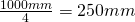# How to convert diopters to focal length?

A lens of focal length f has the “optical power”=As the focal length is given in mm, the optical power is measured in units of. Converting the optical power to units ofresults in “diopters”.

A lens of 4 diopters has a optical power of.
So it has a focal length of.
Correspondingly a lens of f=100mm has an optical power of